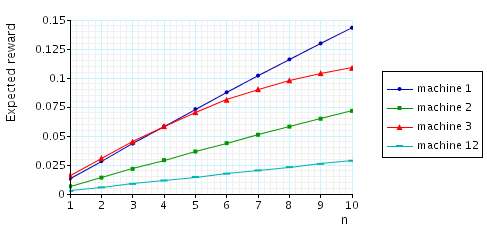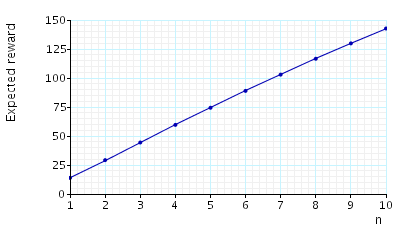# Flexible Manufacturing System

(Ciardo & Trivedi)

### Introduction:

This case study is based on the flexible manufacturing system of [CT93]. The model is represented as a CTMC. We use N to denote the number of tokens in the system. The PRISM source code for this case study given below.

```// flexible manufacturing system [CT93]
// gxn/dxp 11/06/01

ctmc

const int n; // number of tokens

// rates from Pi equal #(Pi) * min(1, np/r)
// where np = (3n)/2 and r = P1+P2+P3+P12
const int np=floor((3*n)/2);
formula r = P1+P2+P3+P12;

module machine1

P1 : [0..n] init n;
P1wM1 : [0..n];
P1M1 : [0..3];
P1d : [0..n];
P1s : [0..n];
P1wP2 : [0..n];
M1 : [0..3] init 3;

[t1] (P1>0) & (M1>0) & (P1M1<3)  -> P1*min(1,np/r) : (P1'=P1-1) & (P1M1'=P1M1+1) & (M1'=M1-1);
[t1] (P1>0) & (M1=0) & (P1wM1<n) -> P1*min(1,np/r) : (P1'=P1-1) & (P1wM1'=P1wM1+1);

[] (P1M1>0) & (P1wM1=0) & (M1<3) & (P1s<n) -> 0.2*P1M1 : (P1M1'=P1M1-1) & (M1'=M1+1) & (P1s'=P1s+1);
[] (P1M1>0) & (P1wM1>0) & (P1s<n) -> 0.2*P1M1 : (P1wM1'=P1wM1-1) & (P1s'=P1s+1);

[] (P1M1>0) & (P2wP1=0) & (P1wM1=0) & (M1<3) & (P1wP2<n) -> 0.05*P1M1 : (P1M1'=P1M1-1) & (M1'=M1+1) & (P1wP2'=P1wP2+1);
[] (P1M1>0) & (P2wP1=0) & (P1wM1>0) & (P1wP2<n) -> 0.05*P1M1 : (P1wM1'=P1wM1-1) & (P1wP2'=P1wP2+1);

[p1p2] (P1M1>0) & (P2wP1>0) & (P1wM1=0) & (M1<3) -> 0.05*P1M1 : (P1M1'=P1M1-1) & (M1'=M1+1);
[p1p2] (P1M1>0) & (P2wP1>0) & (P1wM1>0) -> 0.05*P1M1 : (P1wM1'=P1wM1-1);

[p1p2] (P1wP2>0)  -> 1: (P1wP2'=P1wP2-1);
[]     (P1s>0) & (P1+P1s<=n) -> 1/60 : (P1s'=0) & (P1'=P1+P1s);
[fp12] (P1+P12s<=n) -> 1: (P1'=P1+P12s);

endmodule

module machine2

P2 : [0..n] init n;
P2wM2 : [0..n];
P2M2 : [0..1];
P2s : [0..n];
P2wP1 : [0..n];
M2 : [0..1] init 1;

[t2] (P2>0) & (M2>0) & (P2M2<1)  -> P2*min(1,np/r) : (P2'=P2-1) & (P2M2'=P2M2+1) & (M2'=M2-1);
[t2] (P2>0) & (M2=0) & (P2wM2<n) -> P2*min(1,np/r) : (P2'=P2-1) & (P2wM2'=P2wM2+1);

[] (P2M2>0) & (P2wM2=0) & (M2<1) & (P2s<n) -> 0.1 : (P2M2'=P2M2-1) & (M2'=M2+1) & (P2s'=P2s+1);
[] (P2M2>0) & (P2wM2>0) & (P2s<n) -> 0.1 : (P2wM2'=P2wM2-1) & (P2s'=P2s+1);

[] (P2M2>0) & (P1wP2=0) & (P2wM2=0) & (M2<1) & (P2wP1<n) -> 1/15: (P2M2'=P2M2-1) & (M2'=M2+1) & (P2wP1'=P2wP1+1);
[] (P2M2>0) & (P1wP2=0) & (P2wM2>0) & (P2wP1<n) -> 1/15: (P2wM2'=P2wM2-1) & (P2wP1'=P2wP1+1);

[p1p2] (P2M2>0) & (P1wP2>0) & (P2wM2=0) & (M2<1) -> 1/15: (P2M2'=P2M2-1) & (M2'=M2+1);
[p1p2] (P2M2>0) & (P1wP2>0) & (P2wM2>0) -> 1/15: (P2wM2'=P2wM2-1);

[p1p2] (P2wP1>0) -> 1 : (P2wP1'=P2wP1-1);
[]     (P2s>0) & (P2+P2s<=n) -> 1/60 : (P2s'=0) & (P2'=P2+P2s);
[fp12] (P2+P12s<=n) -> 1 : (P2'=P2+P12s);
[p2p3] (M2>0) -> 1 : (M2'=M2);

endmodule

module machine3

P3 : [0..n] init n;
P3M2 : [0..n];
P3s : [0..n];

[t3] (P3>0) & (P3M2<n) -> P3*min(1,np/r) : (P3'=P3-1) & (P3M2'=P3M2+1);

[p2p3] (P3M2>0) & (P3s<n) -> 1/2 : (P3M2'=P3M2-1) & (P3s'=P3s+1);
[]     (P3s>0) & (P3+P3s<=n) -> 1/60 : (P3s'=0) & (P3'=P3+P3s);

endmodule

module machine12

P12 : [0..n];
P12wM3 : [0..n];
P12M3 : [0..2];
P12s : [0..n];
M3 : [0..2] init 2;

[p1p2] (P12<n) -> 1: (P12'=P12+1);

[t12] (P12>0) & (M3>0) & (P12M3<2) -> P12*min(1,np/r) : (P12'=P12-1) & (P12M3'=P12M3+1) & (M3'=M3-1);
[t12] (P12>0) & (M3=0) & (P12wM3<n) -> P12*min(1,np/r) : (P12'=P12-1) & (P12wM3'=P12wM3+1);

[] (P12M3>0) & (P12wM3=0) & (P12s<n) & (M3<2) -> P12M3 : (P12M3'=P12M3-1) & (P12s'=P12s+1) & (M3'=M3+1);
[] (P12M3>0) & (P12wM3>0) & (P12s<n) -> P12M3 : (P12wM3'=P12wM3-1) & (P12s'=P12s+1);

[fp12] (P12s>0) -> 1/60 : (P12s'=0);

endmodule

// reward structures

// throughput of machine1
rewards "throughput_m1"
[t1]  true : 1;
endrewards
// throughput of machine2
rewards "throughput_m2"
[t2]  true : 1;
endrewards
// throughput of machine3
rewards "throughput_m3"
[t3]  true : 1;
endrewards
// throughput of machine12
rewards "throughput_m12"
[t12]  true : 1;
endrewards
// productivity of the system
rewards "productivity"
[t1]  true : 400;
[t2]  true : 600;
[t3]  true : 100;
[t12] true : 1100;
endrewards
```

### Model Statistics

The table below shows statistics for each model we have built. The first section gives information about the CTMC representing the model: the number of states and the number of non zeros (transitions). The second part refers to the MTBDD which represents this CTMC: the total number of nodes, the number of leaves (terminal nodes), and the amount of memory needed to store it. The last column gives the amount of memory a sparse matrix would need to represent the same CTMC.

 N: Model: MTBDD: Sparse: States: NNZ: Nodes: Leaves: Kb: Kb: 1 54 155 783 9 15.3 2.03 2 810 3,699 4,371 14 85.4 46.5 3 6,520 37,394 10,896 22 212 463 4 35,910 237,120 28,470 30 556 2,919 5 152,712 1,111,482 50,877 43 992 13,621 6 537,768 4,205,670 78,125 53 1,526 51,385 7 1,639,440 13,552,968 120,834 71 2,360 165,227 8 4,459,455 38,533,968 215,250 84 4,204 468,989 9 11,058,190 99,075,405 309,067 108 6,032 1,204,235 10 25,397,658 234,523,289 406,018 126 7,930 2,847,529

### Model Construction Times

The table below gives the times taken to construct the models. This process involves first building a CTMC (represented as an MTBDD) from the system description (in our module based language), and then computing the reachable states using a BDD based fixpoint algorithm. The total time required and the number of fixpoint iterations are given below.

 N: Construction: Time (s): Iter.s: 1 0.173 9 2 0.449 17 3 0.829 25 4 2.62 33 5 5.25 41 6 14.2 49 7 35.8 57 8 44.2 65 9 61.0 73 10 93.7 81

### Model Checking

We now report on the model checking results obtained using PRISM when verifying the following CSL properties.

```// expected throughput of each machine
R{"throughput_m1"}=?  [ S ]
R{"throughput_m2"}=?  [ S ]
R{"throughput_m3"}=?  [ S ]
R{"throughput_m12"}=? [ S ]

// expected productivity of the system
R{"productivity"}=? [ S ]
```

All experiments were carried out on a 2.80GHz PC with 1 Gb memory running Linux and in all iterative methods we stop iterating when the relative error between subsequent iteration vectors is less than 1e-6, that is:The table below gives the times taken to compute the steady state probabilities using both the Jacobi and (backwards) Gauss Seidel methods. The number of iterations required and the time taken using the MTBDD, sparse and hybrid engines is as follows.

 N: Jacobi: Gauss Seidel: Iterations: Time per iteration: (s) Iterations: Time per iteration: (s) MTBDD Sparse Hybrid Sparse Hybrid 1 434 0.004788 < 0.00001 0.000005 75 < 0.00001 < 0.00001 2 378 0.154611 0.000032 0.000040 92 0.000043 0.000087 3 554 1.626347 0.000343 0.000388 106 0.000557 0.000943 4 729 - 0.001771 0.003660 118 0.002610 0.005992 5 905 - 0.008700 0.023281 127 0.011992 0.027898 6 1,080 - 0.033600 0.084465 136 0.044419 0.104735 7 1,258 - 0.109400 0.260022 144 0.143736 0.331993 8 1,438 - 0.325950 0.887426 151 0.420152 0.949457 9 1,619 - 0.865450 3.712932 159 1.077013 2.335075 10 1,804 - - 10.00491 166 - 5.505777

The graphs below present the results obtained for the different CSL properties.

• The expected throughput of each machine:• The expected productivity of the system: